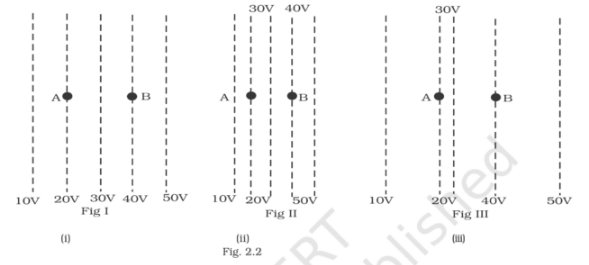# Figure shows some equipotential lines distributed`
Question:

Figure shows some equipotential lines distributed in space. A charged object is moved from point A to point B.(a) the work done in fig (i) is the greatest

(b) the work done in fig (ii) is least

(c) the work done is the same in fig (i), fig (ii), and fig (iii)

(d) the work done in fig (iii) is greater than fig (ii) but equal to that in fig (i)

Solution:

The correct answer is (c) the work done is the same in fig (i), fig (ii), and fig (iii)